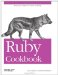# Counting the Days Since an Arbitrary Date

Problem

You want to see how many days have elapsed since a particular date, or how many remain until a date in the future.

Solution

Subtract the earlier date from the later one. If you're using Time objects, the result will be a floating-point number of seconds, so divide by the number of seconds in a day:

```	def last_modified(file)
t1 = File.stat(file).ctime
t2 = Time.now
elapsed = (t2-t1)/(60*60*24)
days ago."
end

last_modified("/etc/passwd")
last_modified("/home/leonardr/")
```

If you're using DateTime objects, the result will be a rational number. You'll probably want to convert it to an integer or floating-point number for display:

```	require 'date'
christmas = DateTime.new(date.year, 12, 25)
christmas = DateTime.new(date.year+1, 12, 25) if date > christmas
difference = (christmas-date).to_i
if difference == 0
puts "Today is Christmas."
else
puts "Only #{difference} day#{"s" unless difference==1} until Christmas."
end
end

# Only 1 day until Christmas.
# Today is Christmas.
# Only 364 days until Christmas.
```

Discussion

Since times are stored internally as numbers, subtracting one from another will give you a number. Since both numbers measure the same thing (time elapsed since some "time zero"), that number will actually mean something: it'll be the number of seconds or days that separate the two times on the timeline.

Of course, this works with other time intervals as well. To display a difference in hours, for Time objects divide the difference by the number of seconds in an hour (3,600, or 1.hour if you're using Rails). For DateTime objects, divide by the number of days in an hour (that is, multiply the difference by 24):

```	sent = DateTime.new(2006, 10, 4, 3, 15)
received = DateTime.new(2006, 10, 5, 16, 33)
puts "You responded to my email #{elapsed.to_f} hours after I sent it."
# You responded to my email 37.3 hours after I sent it.
```

You can even use divmod on a time interval to hack it down into smaller and smaller pieces. Once when I was in college, I wrote a script that displayed how much time remained until the finals I should have been studying for. This method gives you a countdown of the days, hours, minutes, and seconds until some scheduled event:

```	require 'date'
def remaining(date, event)
intervals = [["day", 1], ["hour", 24], ["minute", 60], ["second", 60]]
elapsed = DateTime.now - date
tense = elapsed > 0 ? "since" : "until"
interval = 1.0
parts = intervals.collect do |name, new_interval|
interval /= new_interval
number, elapsed = elapsed.abs.divmod(interval)
"#{number.to_i} #{name}#{"s" unless number == 1}"
end
puts "#{parts.join(", ")} #{tense} #{event}."
end

remaining(DateTime.new(2006, 4, 15, 0, 0, 0, DateTime.now.offset),
# 27 days, 4 hours, 16 minutes, 9 seconds until the book deadline.
remaining(DateTime.new(1999, 4, 23, 8, 0, 0, DateTime.now.offset),
"the Math 114A final")
# 2521 days, 11 hours, 43 minutes, 50 seconds since the Math 114A final.
```

• Recipe 3.5, "Doing Date Arithmetic"Ruby Cookbook (Cookbooks (OReilly))
ISBN: 0596523696
EAN: 2147483647
Year: N/A
Pages: 399